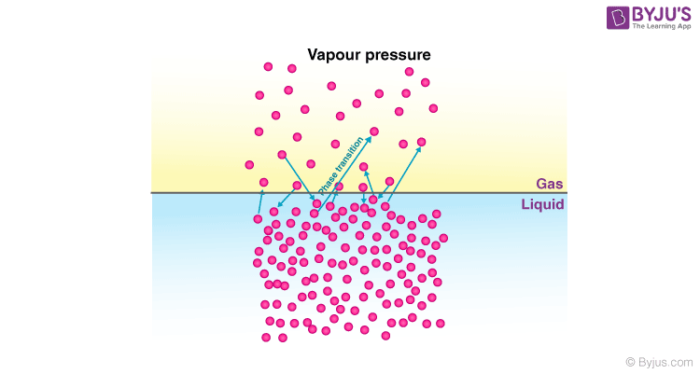Checkout JEE MAINS 2022 Question Paper Analysis : Checkout JEE MAINS 2022 Question Paper Analysis :

# Colligative Properties : Relative Lowering Of Vapour Pressure

Let us first discuss the meaning of colligative properties. According to the general definition, those properties of a solution that depend on the number of solute molecules, irrespective of their nature, with respect to the total number of molecules present in the solution are called colligative properties.

For a solution, relative lowering of vapour pressure, depression of freezing point, elevation in boiling point and osmotic pressure are colligative properties that find great application in our day-to-day lives.

## Relative Lowering of Vapour Pressure

Vapour pressure is the pressure exerted by the vapours over the liquid under the equilibrium conditions at a given temperature. Now let us take an example of a pure liquid, the surface of the liquid is occupied by the molecules of the liquid. Suppose a non-volatile solute is now added to this pure liquid. Since the solute molecules are non-volatile, the vapour above the solution consists of only solvent (pure liquid) molecules. After adding the solute, the vapour pressure of the solution is found to be lower than that of the pure liquid at a given temperature.This lowering in vapour pressure is due to the fact that after the solute was added to the pure liquid (solvent), the liquid surface now had molecules of both, the pure liquid and the solute. The number of solvent molecules escaping into vapour phase gets reduced and as a result the pressure exerted by the vapour phase is also reduced. This is known as relative lowering of vapour pressure. This decrease in vapour pressure depends on the amount of non-volatile solute added in the solution irrespective of its nature and hence it is one of the colligative properties.

### Mathematical Representation

We will now see and understand how this lowering in vapour pressure is determined mathematically.

Let us assume a binary solution in which the mole fraction of the solvent be x1 and that of the solute be x2, p1 be the vapour pressure of the solvent and p1o be the vapour pressure of the solvent in pure state.

According to Raoult’s Law:

p1=x1p1o…………………………..(1)

The decrease in vapour pressure of the solvent (∆p1) is given by:

=> ∆p1=p1o-p1

=> ∆p1=p1o-p1ox1                              [using equation (1)]

=> ∆p1=p1o (1-x1)

Since we have assumed the solution to be binary solution, x2=1-x1

=> ∆p1=p1ox2

=> x2= ∆p1/p1o

The above equation gives the relative lowering in vapour pressure, which is equal to the mole fraction of the solute.

## Frequently Asked Questions – FAQs

### Is Osmosis a Colligative property?

The osmotic pressure of a solution is measured using a semipermeable membrane, a barrier having holes tiny enough to enable solvent molecules to flow through but not solute molecules or ions.

### Is colour a Colligative property?

The colour of a solution is another non-colligative characteristic. In contrast to the colourless salt and sugar solutions, a 0.5 M solution of CuSO4 is vivid blue. Viscosity, surface tension, and solubility are examples of non-colligative characteristics.

### What is Colligative property and its types?

The concentration of solute molecules or ions, but not the identity of the solute, determines the colligative characteristics of solutions. Vapour pressure reduction, boiling point elevation, freezing point depression, and osmotic pressure reduction are all colligative characteristics.

### What is a non-volatile solute?

Under current conditions, a non-volatile material is one that does not quickly evaporate into a gas. Non-volatile compounds have a high boiling point and a low vapour pressure. Non-volatile solutes include things like sugar and salt. Alcohol, mercury, and gasoline are examples of volatile chemicals.

### How Colligative properties are commonly used in everyday life?

We employ freezing point depression as a colligative characteristic in our daily lives. Many antifreezes used in vehicle radiators have a lower freezing point than standard antifreezes, allowing automobile engines to run in sub freezing weather.

Test your Knowledge on Colligative properties relative lowering of vapour pressure!

1. Vidisha Rathi